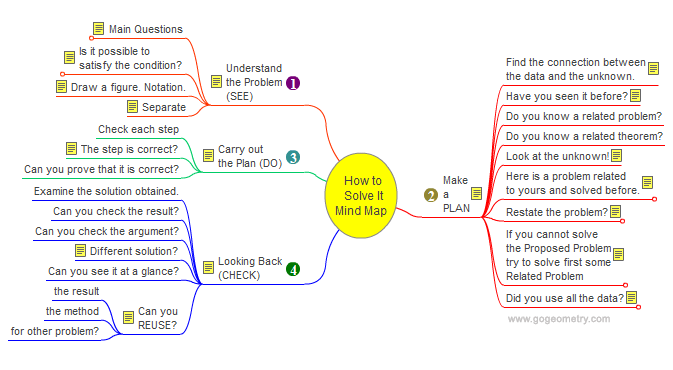Problem 492. Cyclic Quadrilateral, Circle, Perpendicular, Parallel. Level: High School, College, SAT Prep. The figure shows a cyclic quadrilateral ABCD and a circle O. AEF is perpendicular to AC (E on the circle O and F on DB extended), DGH is perpendicular to BD (G on the circle O and H on AC extended), Prove that FH, BC, and EG are parallel. Cyclic quadrilateral is a quadrilateral whose vertices all lie on a single circle.How to Solve It, Mind Map George Pólya's 1945 book "How to Solve It, A new aspect of Mathematical Method", is a book describing methods of problem solving. It suggests the following steps when solving a mathematical problem: (1) First, you have to understand the problem. (2) After understanding, then make a plan. (3) Carry out the plan. (4) Look back on your work. How could it be better?Recent Additions